Human 2D pose estimation is the problem of localizing human body parts such as the shoulders, elbows and ankles from an input image or video. In most of today’s real world application of human pose estimation, a high degree of accuracy as well as “real-time” inference is required.

OpenPose, developed by researchers at the Carnegie Mellon University can be considered as the state of the art approach for real-time human pose estimation. The code base is open-sourced on github and is very well documented. Openpose is originally written in C++ and Caffe.

Throughout the article, I may also be referencing some code from here , an accurate Tensorflow implementation of OpenPose. Use this article as a starting point and read the full paper afterwards as I have left out some specific details from the paper to conserve space.

This article is split into three different parts. The first part will analyze the overall setup of OpenPose; the main neural network architecture and common notations used throughout the paper. The second part will go into detail regarding confidence maps and part affinity maps. The third part of the article will discuss how the key points are finally assembled correctly from the outputs of the neural network by viewing the problem as a Graph matching problem.

Before we go into the details of OpenPose, it is worth noting that there exists two versions of the paper this and this. The first original paper was submitted on 24 Nov 2016 and the most recent one was submitted on 18 Dec 2018. There are a couple minor differences such as the neural network architecture and some post processing aspect resulting in an improved speed and accuracy. However, the general idea and overall pipeline is still the same. For more details on the differences, you can find it in the 1st section of the most recent paper here. In this article, we will explore the original version of the paper since at the time of writing this article, most implementations on github are still using the steps described in the first paper.

### Overall Pipeline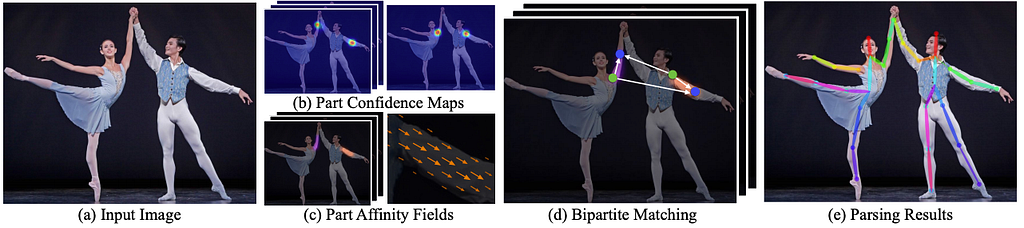Fig 1. Overall Pipeline. Image taken from “Realtime Multi-Person 2D Pose Estimation using Part Affinity Fields”.

The pipeline from OpenPose is actually pretty simple and straightforward.

First, an input RGB image (Fig 1a) is fed as input into a “two-branch multi-stage” CNN. Two branch means that the CNN produces two different outputs. Multi-stage simply means that the network is stacked one on top of the other at every stage. (This step is analogous to simply increasing the depth of the neural network in order to capture more refined outputs towards the latter stages.)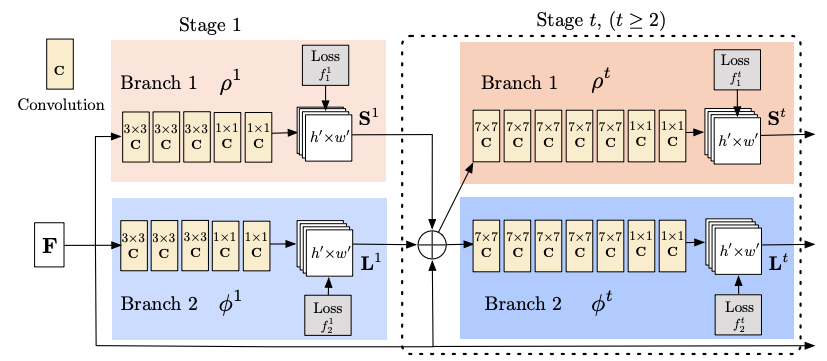Fig 2. Architecture of the two-branch multi-stage CNN. Image taken from “Realtime Multi-Person 2D Pose Estimation using Part Affinity Fields”.

Two-branches: The top branch, shown in beige, predicts the confidence maps (Fig 1b) of different body parts location such as the right eye, left eye, right elbow and others. The bottom branch, shown in blue, predicts the affinity fields (Fig 1c), which represents a degree of association between different body parts.

Multi-Stage: At the first stage (left half of Fig 2), the network produces an initial set of detection confidence maps S and a set of part affinity fields L. Then, in each subsequent stages (right half of Fig 2), the predictions from both branches in the previous stage, along with the original image features F, are concatenated (represented by the + sign in Fig 2) and used to produce more refined predictions. In the OpenPose implementation, the final stage t is chosen to be 6.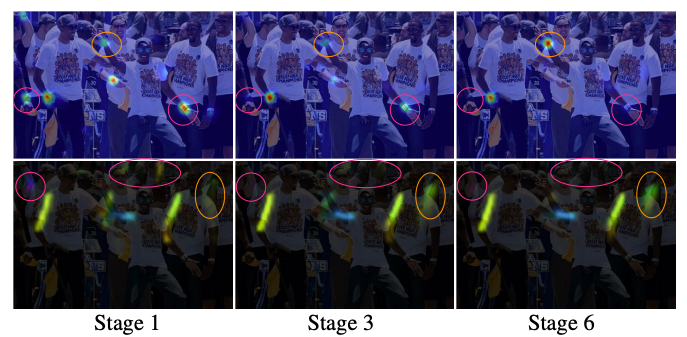Fig 3. Outcome of a multi-stage network. The TOP row shows the network predicting confidence maps of the right wrist while the BOTTOM row shows the network predicting the Part Affinity Fields of right forearm (right shoulder — right wrist) across different stages.

Fig 3 shows the positive benefits of a multi-stage setup. In the example, we observe that there are some initial confusion between the left and right body parts in the first few stages. But as the stage progresses, the network becomes better at making those distinctions.

Finally, the confidence maps and affinity fields are being processed by greedy inference (Fig 1d) to output the 2D key points for all people in the image (Fig 1e).

### Confidence Maps

Referring back to Fig 2, the top branch of the neural network produces a set of detection confidence maps S. This is mathematically defined as follows.

J, the total number of body parts, depends on the dataset that OpenPose is trained with. For COCO dataset, J = 19 since there are 18 different body keypoints + 1 background. The figure below shows the different body parts with its assigned ID for the COCO dataset.

To better understand what set S represents, consider this example. For model trained with the COCO dataset, the set S will have elements of S1, S2, S3,…, S19. For this example, let’s assume that the element S1 corresponds to the confidence map for the key point id of 0 (in Fig 5) which refers to the nose. Then, the confidence map might look as follows.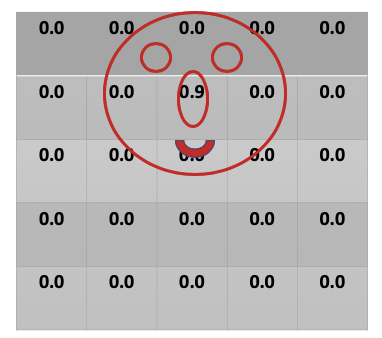Fig 6. A very simplified diagram showing a single confidence map where each cell in the table corresponds to a pixel in the original image of dimensions w x h. The value in each cell represents the confidence that a Nose is present.

In Fig 6, we assume that the full picture has a width and height of 5, resulting in a 5 X 5 confidence map. In this example, there is only one face in the picture. Hence, for the confidence map S1 (predicts confidence in detecting nose) , we only see an area of high confidence,0.9 ,in the region where there is a nose.

### Part Affinity Field (PAF) Maps

Referring back to Fig 2, the bottom branch of the neural network produces a set of part affinity field maps L. This is mathematically defined as follows.

C, the total number of limbs, depends on the dataset that OpenPose is trained with. The paper refer to part pairs as limbs for clarity, despite the fact that some body part pairs are not human limbs. For COCO dataset, C = 19. The figure below shows the different part pairs.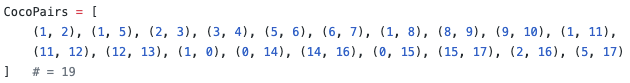Fig 8. An array of tuples. Each tuple pair represents body part ID pairs.

You can imagine that each element in the set L is a map of size w x h where each cell contains a 2d vector representing the direction of pair elements. For example in Fig 1c, the body part pair consists of the right shoulder to the right elbow. The diagram then shows a directional vector which points from the right shoulder to right elbow.

Now that we have a better understanding of the mathematical notations and what it represents, we can move on to the next section.

### Neural Network Details

The image is first analyzed by a pre-trained convolutional neural network such as the first 10 layers of VGG-19, to produce a set of feature maps F. This choice of feature extractor to produce F is not limited to VGG-19. There are other variations of OpenPose that uses Mobilenet or Resnet to extract the image features before passing it to the rest of the neural network shown in Fig 2.

Stage 1: the network produces a set of detection confidence maps S and a set of part affinity fields L. The symbol 𝛒 is used as a function variable which represents the CNN with input F to produce the output map S. The symbol 𝛟 is used as a function variable which represents the CNN with input F to produce the output map L. The annotation “1” at the top of each symbol means inference at the first stage.

Stage t: the predictions from both branches in the previous stage, along with the original image features , are concatenated and used to produce more refined predictions.

In the OpenPose paper, t goes from 2 to 6. The comma in the above figure represents concatenation between maps.

Loss Functions: In order for the network to learn how to generate the best sets of S and L, the authors applies two loss functions at the end of each stage, one at each branch respectively. The paper uses a standard L2 loss between the estimated predictions and ground truth maps and fields. (We will later also see how the authors have come up a way to create the ground truth maps for each S and L). Moreover, the authors has added some weight to the loss functions to address a practical issue that some datasets do not completely label all people. The loss functions at a particular stage t are given as follows.

1. The notation p represents a single pixel location in a w x h image.
2. The * notation next to the set S and L means that it is the ground truth
3. The output of S(p) is a 1 dimensional vector which consists of the confidence score for that particular body part j at image location p.
4. The output of L(p) is a 2 dimensional vector which consists of the directional vector for that particular limb c at image location p.
5. In the OpenPose paper, J , the total number of body part is 19. Also, C , the total number of “limbs” or body to body connections is 19.
6. W(p) represents the weighing function as previously mentioned. W(p) = 0 when the annotation is missing at an image location p. The mask is used to avoid penalizing the true positive predictions during training.

Overall Loss Function: Finally, combining the two loss functions we come up with the overall objective.

### Neural Network Implementation (in Caffe)

The authors of OpenPose uses Caffe to implement the neural network. Don’t worry if you are not familiar with Caffe, like many other deep learning frameworks, Caffe is very intuitive and easy to understand. Caffe models are defined in .prototxt files. Below is a truncated version of the neural network model defined using Caffe. The full model file takes a lot of space and hence I have decided to just show the first few lines. You can find here for the full model definition.

In order to better visualize the neural network architecture, we use a network visualization tool like https://ethereon.github.io/netscope/quickstart.html where it converts texts into some visualization which is easier to understand. I recommend you to try it out yourself as it has some interactivity where you can hover and it will show you more details of each module.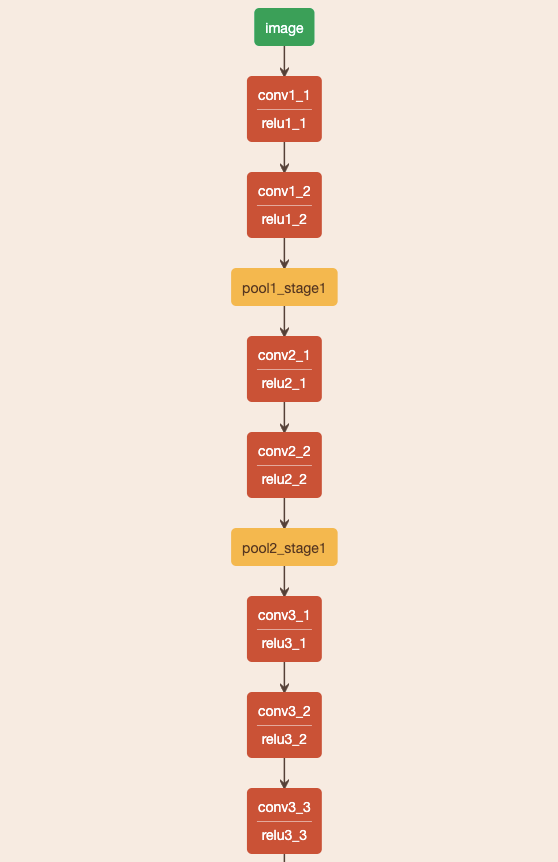Fig 9. A snapshot of the first few layers of the openpose neural network. This section corresponds to part of the neural network where it is trying to generate the set of feature maps F.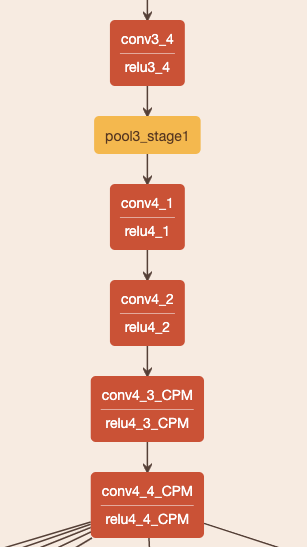Fig 10. A snapshot of the first few layers of the openpose neural network, continuation from Fig 9. This section corresponds to part of the neural network where it is trying to generate the set of feature maps F.

An important point to note here is that the output of the module “relu4_4_CPM” is the set of image features F described in the paper (Fig 2). This set of image features F is concatenated along with predictions from both branches shown in Fig 2 to produce more refined predictions in later stages.

### Stage 1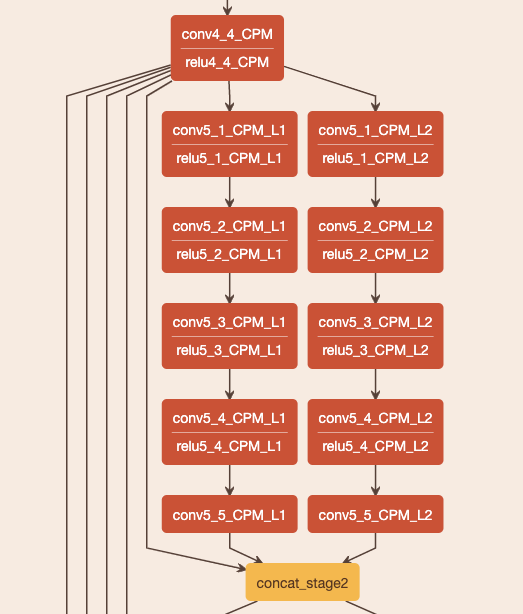Fig 11. A snapshot of the first stage of the neural network.

As shown in Fig 11, you can see that the output from the module “relu4_4_CPM” is being fed into two modules, “conv5_1_CPM_L1” and “conv5_1_CPM_L2”. The first module correspond to the BOTTOM branch (predicting the set of PAF vectors) of Fig 2, while the second module correspond to the TOP branch (predicting the set of confidence vectors) of Fig 2. The output dimension of “conv5_5_CPM_L2” is (w x h x 19) where 19 corresponds to the 19 different keypoints in the COCO dataset. The output dimension of “conv5_5_CPM_L1” is (w x h x 38) where 38 = 19 * 2 corresponds to the 19 different “limbs” defined in the COCO dataset. And is multiplied by 2 since each cell in the map of each limb represents a vector which has an x & y value.

### Stage t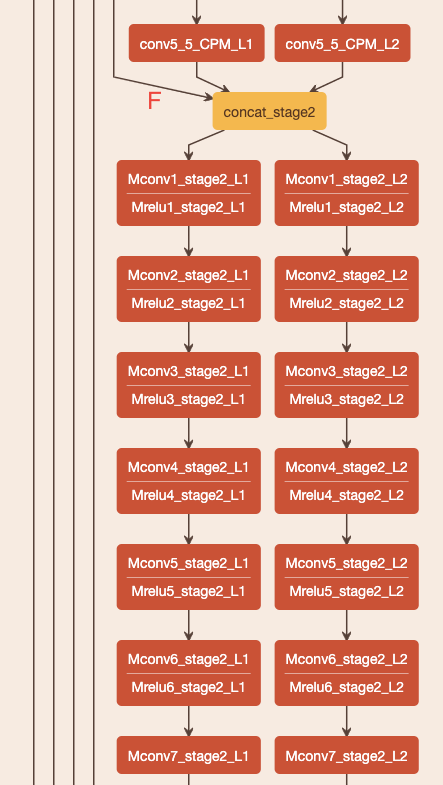Fig 12. A snapshot of the second stage of the neural network.

As shown in Fig 12 , an important note here is that the concatenation stage takes three input. That is F and the output from the first stage S and L. This is then fed again into the two different branches. This process repeats until t = 6 where it finally returns the most refined values.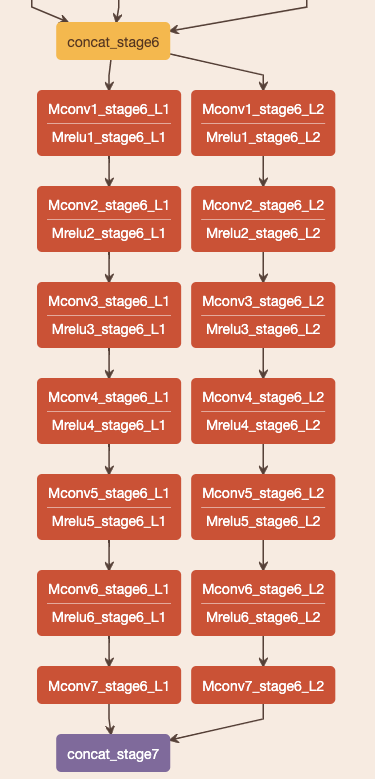Fig 13. A snapshot of the FINAL stage, stage 6, of the neural network.

The final outputs are then concatenated and returned for greedy matching discussed in the next few parts of the article.